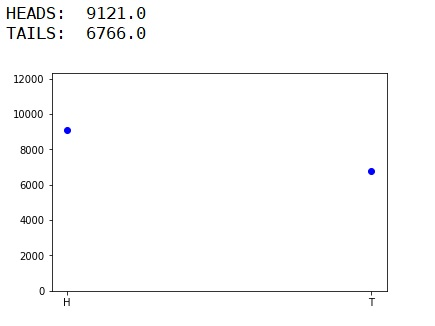# Python program for biased coin flipping simulation

Biased coin flipping in Python: Here, we are going to learn how to simulate the occurrence coin face i.e. H - HEAD, T – TAIL in Python?
Submitted by Anuj Singh, on July 31, 2019

Here, we will be simulating the occurrence coin face i.e. H - HEAD, T - TAIL. Simply we are going to use an inbuilt library called as random to call a random value from given set and thereby we can stimulate the occurrence value by storing the occurrence in the list ls of length 2 representing each face of the coin as ls[] represents the occurrence of:

Here, we will be stimulating the occurrence of each dice face i.e. 1, 2, 3, 4, 4, 4, 5, 6, 6, 6, 6. Simply we are going to use an inbuilt library called as random to call a random value from given set and thereby we can stimulate the occurrence value by storing the occurrence in the list ls of length 6 representing each face of the dice as ls represents the occurrence of face 5.

```    ls - coin(H)
ls - coin(T)
```

Then using the library pylab, we can plot the value of each occurrence and can stimulate it.

The deviation is clear that each of the faces i.e. heads and tails have an unequal probability of occurrence.

Program:

```import random
import pylab as py

def flip():
return random.choice(['H','H','H','T','T','H','T'])

ls = [0,0]
chance = [104, 203, 302, 401, 505, 646, 756, 855, 985, 4565, 6565]
for n in chance:
for k in range(n):
scr = flip()
if scr == 'H':
ls = ls + 4/4
else:
ls = ls + 4/4

py.figure()
py.plot(['H','T'], ls, 'bo')
py.ylim(0,12300)

print("TAILS: ", ls)
```

OutputPreparation